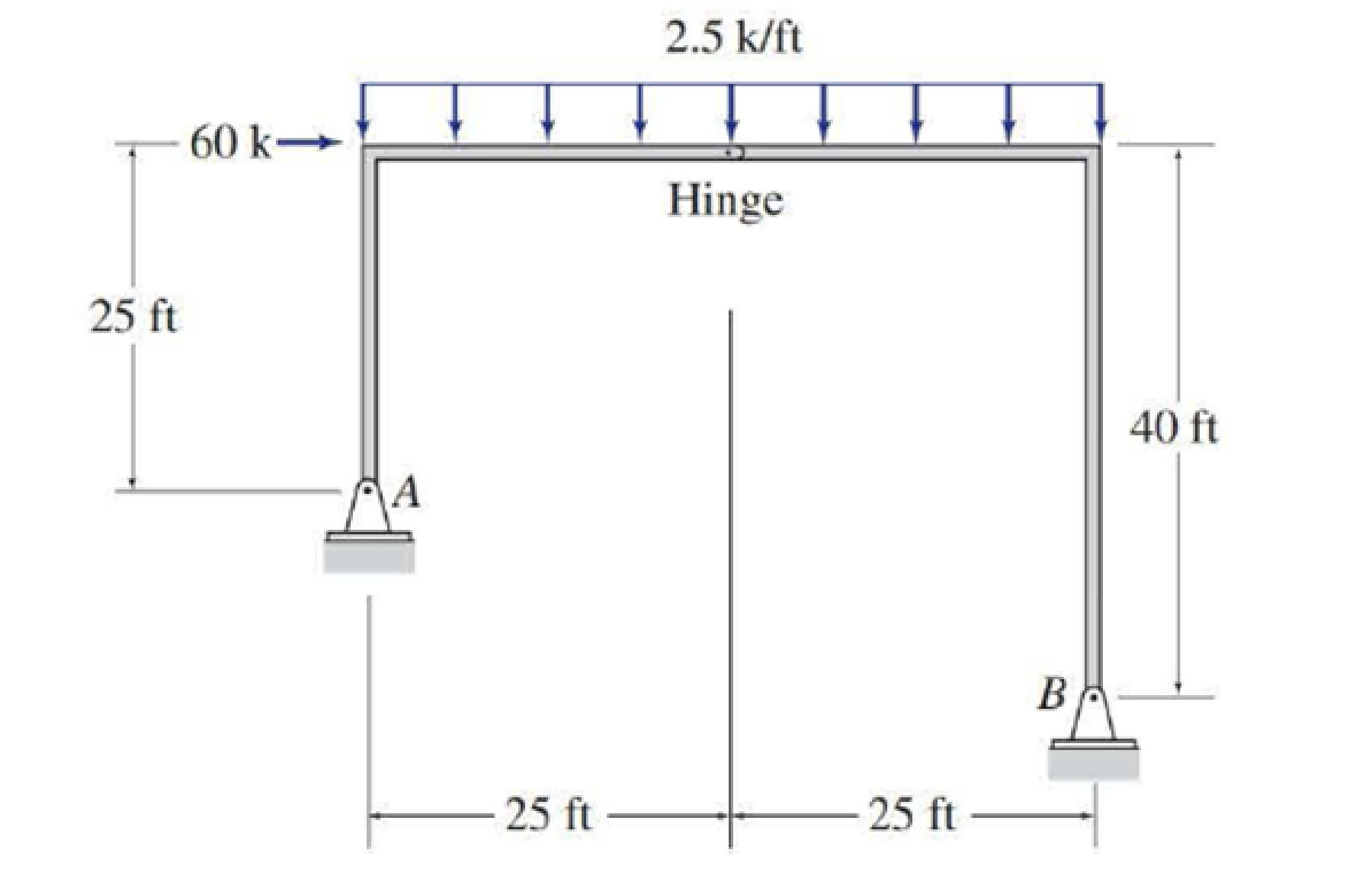# Determine the reactions at the supports for the structures shown. FIG.P3.40

#### Solutions

Chapter
Section
Chapter 3, Problem 40P
Textbook Problem
27 views

## Determine the reactions at the supports for the structures shown.FIG.P3.40

To determine

Calculate the support reactions for the given structure.

### Explanation of Solution

Given information:

The structure is given in the Figure.

Apply the sign conventions for calculating reaction forces and moments using the three equations of equilibrium as shown below.

• For summation of forces along x-direction is equal to zero (Fx=0), consider the forces acting towards right side as positive (+) and the forces acting towards left side as negative ().
• For summation of forces along y-direction is equal to zero (Fy=0), consider the upward force as positive (+) and the downward force as negative ().
• For summation of moment about a point is equal to zero (Matapoint=0), consider the clockwise moment as negative and the counter clockwise moment as positive.

Calculation:

Let Ax and Ay be the horizontal and vertical reactions at the hinged support A.

Let Bx and By be the horizontal reaction and vertical reaction at the hinged support B.

Sketch the free body diagram of the structure as shown in Figure 1.

Use equilibrium equations:

Summation of moments about A is equal to 0.

MA=060(25)2.5(50)(502)Bx(15)+By(50)=015Bx+50By=4,625 (1)

For the member BC, the summation of moments about C is equal to 0.

MCBC=02.5(25)(252)Bx(40)+By(25)=040Bx+25By=781

### Still sussing out bartleby?

Check out a sample textbook solution.

See a sample solution

#### The Solution to Your Study Problems

Bartleby provides explanations to thousands of textbook problems written by our experts, many with advanced degrees!

Get Started

Find more solutions based on key concepts
What does strain represent?

Engineering Fundamentals: An Introduction to Engineering (MindTap Course List)

What is meant by font style? (166)

Enhanced Discovering Computers 2017 (Shelly Cashman Series) (MindTap Course List)

What is the purpose of an EISP?

Management Of Information Security

What equations describes the income statement?

Cornerstones of Financial Accounting

What file must be accessed in order to analyze SNMP logs?

Network+ Guide to Networks (MindTap Course List)

What determines the ampacity of a wire?

EBK ELECTRICAL WIRING RESIDENTIAL

What are best practices?

Principles of Information Systems (MindTap Course List)

Which law amended the Computer Fraud and Abuse Act of 1986, and what did it change?

Principles of Information Security (MindTap Course List)

The resultant of the three forces is a force R that passes through point B.Â Determine R and F.

International Edition---engineering Mechanics: Statics, 4th Edition

If your motherboard supports ECC DDR3 memory, can you substitute non-ECC DDR3 memory?

A+ Guide to Hardware (Standalone Book) (MindTap Course List)